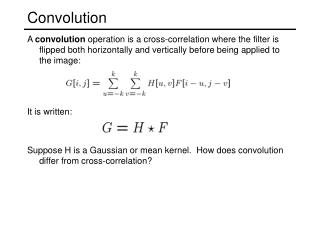DownloadDownload PresentationConvolution

# Convolution

Télécharger la présentation## Convolution

- - - - - - - - - - - - - - - - - - - - - - - - - - - E N D - - - - - - - - - - - - - - - - - - - - - - - - - - -
##### Presentation Transcript

1. Convolution • A convolution operation is a cross-correlation where the filter is flipped both horizontally and vertically before being applied to the image: • It is written: • Suppose H is a Gaussian or mean kernel. How does convolution differ from cross-correlation?

2. Continuous filtering • We can also apply continuous filters to continuous images. • In the case of cross correlation: • In the case of convolution: • Note that the image and filter are infinite.

3. The gradient direction is given by: • how does this relate to the direction of the edge? • The edge strength is given by the gradient magnitude Image gradient • The gradient of an image: • The gradient points in the direction of most rapid change in intensity

4. Effects of noise • Consider a single row or column of the image • Plotting intensity as a function of position gives a signal • Where is the edge?

5. Look for peaks in Solution: smooth first • Where is the edge?

6. Derivative theorem of convolution • This saves us one operation:

7. Laplacian of Gaussian • Consider Laplacian of Gaussian operator • Where is the edge? • Zero-crossings of bottom graph

8. Laplacian of Gaussian • is the Laplacian operator: 2D edge detection filters Gaussian derivative of Gaussian filter demo

9. Edge detection by subtraction original

10. Edge detection by subtraction smoothed (5x5 Gaussian)

11. Edge detection by subtraction Why does this work? smoothed – original (scaled by 4, offset +128) filter demo

12. Gaussian - image filter Gaussian delta function Laplacian of Gaussian